# class TSpectrumTransform: public TNamed

```THIS CLASS CONTAINS ORTHOGONAL TRANSFORM  FUNCTIONS.

These functions were written by:
Miroslav Morhac
Institute of Physics
Dubravska cesta 9, 842 28 BRATISLAVA
SLOVAKIA

email:fyzimiro@savba.sk,    fax:+421 7 54772479

The original code in C has been repackaged as a C++ class by R.Brun

The algorithms in this class have been published in the following
references:

 C.V. Hampton, B. Lian, Wm. C. McHarris: Fast-Fourier-transform
spectral enhancement techniques for gamma-ray spectroscopy.NIM A353
(1994) 280-284.
 Morhac M., Matousek V., New adaptive Cosine-Walsh  transform and
its application to nuclear data compression, IEEE Transactions on
Signal Processing 48 (2000) 2693.
 Morhac M., Matousek V., Data compression using new fast adaptive
Cosine-Haar transforms, Digital Signal Processing 8 (1998) 63.
 Morhac M., Matousek V.: Multidimensional nuclear data compression
using fast adaptive Walsh-Haar transform. Acta Physica Slovaca 51
(2001) 307.

```

## Function Members (Methods)

public:
protected:
 void BitReverse(float* working_space, Int_t num) void BitReverseHaar(float* working_space, Int_t shift, Int_t num, Int_t start) virtual void TObject::DoError(int level, const char* location, const char* fmt, va_list va) const void Fourier(float* working_space, Int_t num, Int_t hartley, Int_t direction, Int_t zt_clear) Int_t GeneralExe(float* working_space, Int_t zt_clear, Int_t num, Int_t degree, Int_t type) Int_t GeneralInv(float* working_space, Int_t num, Int_t degree, Int_t type) void Haar(float* working_space, Int_t num, Int_t direction) void TObject::MakeZombie() void Walsh(float* working_space, Int_t num)

## Data Members

public:
 enum { kTransformHaar kTransformWalsh kTransformCos kTransformSin kTransformFourier kTransformHartley kTransformFourierWalsh kTransformFourierHaar kTransformWalshHaar kTransformCosWalsh kTransformCosHaar kTransformSinWalsh kTransformSinHaar kTransformForward kTransformInverse }; enum TObject::EStatusBits { kCanDelete kMustCleanup kObjInCanvas kIsReferenced kHasUUID kCannotPick kNoContextMenu kInvalidObject }; enum TObject::[unnamed] { kIsOnHeap kNotDeleted kZombie kBitMask kSingleKey kOverwrite kWriteDelete };
protected:
 Int_t fDegree degree of mixed transform, applies only for Fourier-Walsh, Fourier-Haar, Walsh-Haar, Cosine-Walsh, Cosine-Haar, Sine-Walsh, Sine-Haar transforms Int_t fDirection forward or inverse transform Float_t fEnhanceCoeff multiplication coefficient applied in enhanced region; Float_t fFilterCoeff value set in the filtered region TString TNamed::fName object identifier Int_t fSize length of transformed data TString TNamed::fTitle object title Int_t fTransformType type of transformation (Haar, Walsh, Cosine, Sine, Fourier, Hartley, Fourier-Walsh, Fourier-Haar, Walsh-Haar, Cosine-Walsh, Cosine-Haar, Sine-Walsh, Sine-Haar) Int_t fXmax last channel of filtered or enhanced region Int_t fXmin first channel of filtered or enhanced region

## Class Charts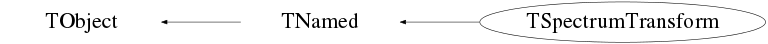## Function documentation

```default constructor
```
TSpectrumTransform(Int_t size)
```the constructor creates TSpectrumTransform object. Its size must be > than zero and must be power of 2.
It sets default transform type to be Cosine transform. Transform parameters can be changed using setter functions.
```

```destructor
```
void Haar(float* working_space, Int_t num, Int_t direction)
```AUXILIARY FUNCION

This function calculates Haar transform of a part of data
Function parameters:
-working_space-pointer to vector of transformed data
-num-length of processed data
-direction-forward or inverse transform

```
void Walsh(float* working_space, Int_t num)
```AUXILIARY FUNCION

This function calculates Walsh transform of a part of data
Function parameters:
-working_space-pointer to vector of transformed data
-num-length of processed data

```
void BitReverse(float* working_space, Int_t num)
```AUXILIARY FUNCION

This function carries out bir-reverse reordering of data
Function parameters:
-working_space-pointer to vector of processed data
-num-length of processed data

```
void Fourier(float* working_space, Int_t num, Int_t hartley, Int_t direction, Int_t zt_clear)
```AUXILIARY FUNCION

This function calculates Fourier based transform of a part of data
Function parameters:
-working_space-pointer to vector of transformed data
-num-length of processed data
-hartley-1 if it is Hartley transform, 0 othewise
-direction-forward or inverse transform

```
void BitReverseHaar(float* working_space, Int_t shift, Int_t num, Int_t start)
```AUXILIARY FUNCION

This function carries out bir-reverse reordering for Haar transform
Function parameters:
-working_space-pointer to vector of processed data
-shift-shift of position of processing
-start-initial position of processed data
-num-length of processed data

```
int GeneralExe(float* working_space, Int_t zt_clear, Int_t num, Int_t degree, Int_t type)
```AUXILIARY FUNCION

This function calculates generalized (mixed) transforms of different degrees
Function parameters:
-working_space-pointer to vector of transformed data
-zt_clear-flag to clear imaginary data before staring
-num-length of processed data
-degree-degree of transform (see manual)
-type-type of mixed transform (see manual)

```
int GeneralInv(float* working_space, Int_t num, Int_t degree, Int_t type)
```AUXILIARY FUNCION

This function calculates inverse generalized (mixed) transforms
Function parameters:
-working_space-pointer to vector of transformed data
-num-length of processed data
-degree-degree of transform (see manual)
-type-type of mixed transform (see manual)

```
void Transform(const float* source, float* destVector)
```        ONE-DIMENSIONAL TRANSFORM FUNCTION
This function transforms the source spectrum. The calling program
should fill in input parameters.
Transformed data are written into dest spectrum.

Function parameters:
source-pointer to the vector of source spectrum, its length should
be size except for inverse FOURIER, FOUR-WALSH, FOUR-HAAR
transform. These need 2*size length to supply real and
imaginary coefficients.
destVector-pointer to the vector of dest data, its length should be
size except for direct FOURIER, FOUR-WALSH, FOUR-HAAR. These
need 2*size length to store real and imaginary coefficients

```

Transform methods

Goal: to analyze experimental data using orthogonal transforms

         orthogonal transforms can be successfully used for the processing of nuclear spectra (not only)

         they can be used to remove high frequency noise, to increase signal-to-background ratio as well as to enhance low intensity components , to carry out e.g. Fourier analysis etc.

         we have implemented the function for the calculation of the commonly used orthogonal transforms as well as functions for the filtration and enhancement of experimental data

Function:

void TSpectrumTransform::Transform(const float *fSource, float *fDest)

This function transforms the source spectrum according to the given input parameters. Transformed data are written into dest spectrum. Before the Transform function is called the class must be created by constructor and the type of the transform as well as some other parameters must be set using a set of setter functions.

Member variables of TSpectrumTransform class:

fSource-pointer to the vector of source spectrum. Its length should be equal to the fSize parameter except for inverse FOURIER, FOUR-WALSH, FOUR-HAAR transforms. These need 2*fSize length to supply real and imaginary coefficients.

fDest-pointer to the vector of destination spectrum. Its length should be equal to the fSize parameter except for inverse FOURIER, FOUR-WALSH, FOUR-HAAR transforms. These need 2*fSize length to store real and imaginary coefficients.

fSize-basic length of the source and dest spectrum. It should be power of 2.

fType-type of transform

Classic transforms:

kTransformHaar

kTransformWalsh

kTransformCos

kTransformSin

kTransformFourier

kTransformHartey

Mixed transforms:

kTransformFourierWalsh

kTransformFourierHaar

kTransformWalshHaar

kTransformCosWalsh

kTransformCosHaar

kTransformSinWalsh

kTransformSinHaar

fDirection-direction-transform direction (forward, inverse)

kTransformForward

kTransformInverse

fDegree-applies only for mixed transforms , , .

Allowed range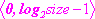.

References:

 C.V. Hampton, B. Lian, Wm. C. McHarris: Fast-Fourier-transform spectral enhancement techniques for gamma-ray spectroscopy. NIM A353 (1994) 280-284.

 Morháč M., Matouek V., New adaptive Cosine-Walsh  transform and its application to nuclear data compression, IEEE Transactions on Signal Processing 48 (2000) 2693.

 Morháč M., Matouek V., Data compression using new fast adaptive Cosine-Haar transforms, Digital Signal Processing 8 (1998) 63.

 Morháč M., Matouek V.: Multidimensional nuclear data compression using fast adaptive Walsh-Haar transform. Acta Physica Slovaca 51 (2001) 307.

Example   script Transform.c: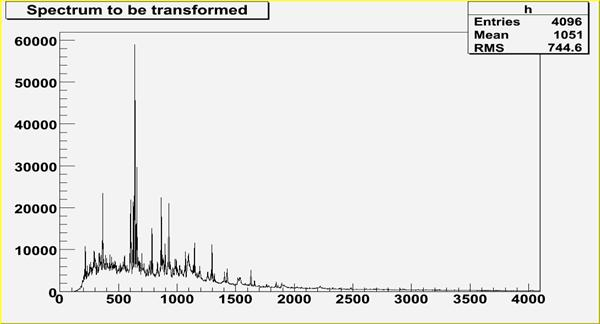Fig. 1 Original gamma-ray spectrum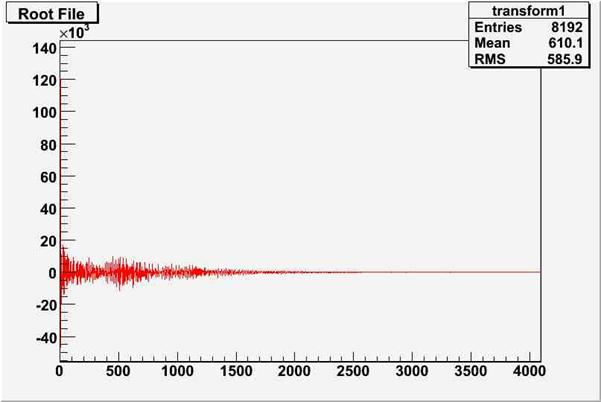Fig. 2 Transformed spectrum from Fig. 1 using Cosine transform

Script:

// Example to illustrate Transform function (class TSpectrumTransform).

// To execute this example, do

// root > .x Transform.C

#include <TSpectrum>

#include <TSpectrumTransform>

void Transform() {

Int_t i;

Double_t nbins = 4096;

Double_t xmin  = 0;

Double_t xmax  = (Double_t)nbins;

Float_t * source = new float[nbins];

Float_t * dest = new float[nbins];

TH1F *h = new TH1F("h","Transformed spectrum using Cosine transform",nbins,xmin,xmax);

TFile *f = new TFile("spectra\\TSpectrum.root");

h=(TH1F*) f->Get("transform1;1");

for (i = 0; i < nbins; i++) source[i]=h->GetBinContent(i + 1);

TCanvas *Transform1 = gROOT->GetListOfCanvases()->FindObject("Transform1");

if (!Transform1) Transform1 = new TCanvas("Transform","Transform1",10,10,1000,700);

TSpectrum *s = new TSpectrum();

TSpectrumTransform *t = new TSpectrumTransform(4096);

t->SetTransformType(t->kTransformCos,0);

t->SetDirection(t->kTransformForward);

t->Transform(source,dest);

for (i = 0; i < nbins; i++) h->SetBinContent(i + 1,dest[i]);

h->SetLineColor(kRed);

h->Draw("L");

}

void FilterZonal(const float* source, float* destVector)
```        ONE-DIMENSIONAL FILTER ZONAL FUNCTION
This function transforms the source spectrum. The calling program
should fill in input parameters. Then it sets transformed
coefficients in the given region (fXmin, fXmax) to the given
fFilterCoeff and transforms it back.
Filtered data are written into dest spectrum.

Function parameters:
source-pointer to the vector of source spectrum, its length should
be size except for inverse FOURIER, FOUR-WALSH, FOUR-HAAR
transform. These need 2*size length to supply real and
imaginary coefficients.
destVector-pointer to the vector of dest data, its length should be
size except for direct FOURIER, FOUR-WALSH, FOUR-HAAR. These
need 2*size length to store real and imaginary coefficients

```

Example of zonal filtering

Function:

void TSpectrumTransform::FilterZonal(const float *fSource, float *fDest)

This function transforms the source spectrum (for details see Transform function). Before the FilterZonal function is called the class must be created by constructor and the type of the transform as well as some other parameters must be set using a set of setter functions. The FilterZonal function sets transformed coefficients in the given region (fXmin, fXmax) to the given fFilterCoeff and transforms it back. Filtered data are written into dest spectrum.

Example  script Filter.c: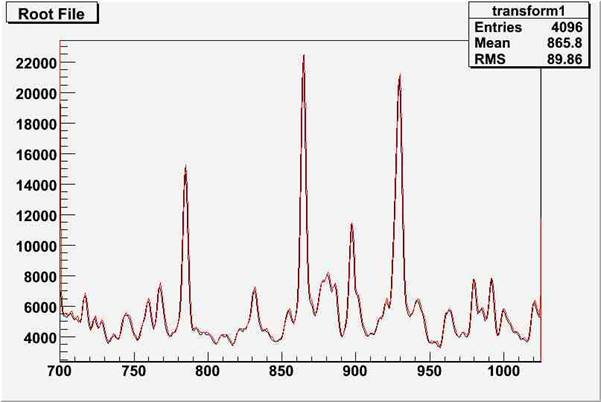Fig. 1 Original spectrum (black line) and filtered spectrum (red line) using Cosine transform and zonal filtration (channels 2048-4095 were set to 0)

Script:

// Example to illustrate FilterZonal function (class TSpectrumTransform).

// To execute this example, do

// root > .x Filter.C

#include <TSpectrum>

#include <TSpectrumTransform>

void Filter() {

Int_t i;

Double_t nbins = 4096;

Double_t xmin  = 0;

Double_t xmax  = (Double_t)nbins;

Float_t * source = new float[nbins];

Float_t * dest = new float[nbins];

TH1F *h = new TH1F("h","Zonal filtering using Cosine transform",nbins,xmin,xmax);

TH1F *d = new TH1F("d","",nbins,xmin,xmax);

TFile *f = new TFile("spectra\\TSpectrum.root");

h=(TH1F*) f->Get("transform1;1");

for (i = 0; i < nbins; i++) source[i]=h->GetBinContent(i + 1);

TCanvas *Transform1 = gROOT->GetListOfCanvases()->FindObject("Transform1");

if (!Transform1) Transform1 = new TCanvas("Transform","Transform1",10,10,1000,700);

h->SetAxisRange(700,1024);

h->Draw("L");

TSpectrum *s = new TSpectrum();

TSpectrumTransform *t = new TSpectrumTransform(4096);

t->SetTransformType(t->kTransformCos,0);

t->SetRegion(2048, 4095);

t->FilterZonal(source,dest);

for (i = 0; i < nbins; i++) d->SetBinContent(i + 1,dest[i]);

d->SetLineColor(kRed);

d->Draw("SAME L");

}

void Enhance(const float* source, float* destVector)
```        ONE-DIMENSIONAL ENHANCE ZONAL FUNCTION
This function transforms the source spectrum. The calling program
should fill in input parameters. Then it multiplies transformed
coefficients in the given region (fXmin, fXmax) by the given
fEnhanceCoeff and transforms it back
Processed data are written into dest spectrum.

Function parameters:
source-pointer to the vector of source spectrum, its length should
be size except for inverse FOURIER, FOUR-WALSh, FOUR-HAAR
transform. These need 2*size length to supply real and
imaginary coefficients.
destVector-pointer to the vector of dest data, its length should be
size except for direct FOURIER, FOUR-WALSh, FOUR-HAAR. These
need 2*size length to store real and imaginary coefficients

```

Example of enhancement

Function:

void TSpectrumTransform::Enhance(const float *fSource, float *fDest)

This function transforms the source spectrum (for details see Transform function). Before the Enhance function is called the class must be created by constructor and the type of the transform as well as some other parameters must be set using a set of setter functions. The Enhance function multiplies transformed coefficients in the given region (fXmin, fXmax) by the given fEnhancCoeff and transforms it back. Enhanced data are written into dest spectrum.

Example   script Enhance.c: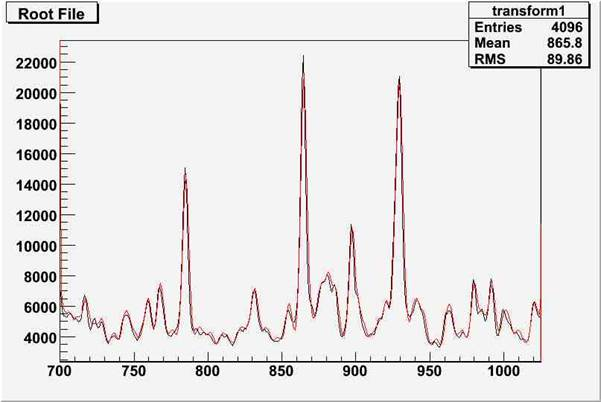Fig. 1 Original spectrum (black line) and enhanced spectrum (red line) using Cosine transform (channels 0-1024 were multiplied by 2)

Script:

// Example to illustrate Enhance function (class TSpectrumTransform).

// To execute this example, do

// root > .x Enhance.C

void Enhance() {

Int_t i;

Double_t nbins = 4096;

Double_t xmin  = 0;

Double_t xmax  = (Double_t)nbins;

Float_t * source = new float[nbins];

Float_t * dest = new float[nbins];

TH1F *h = new TH1F("h","Enhancement using Cosine transform",nbins,xmin,xmax);

TH1F *d = new TH1F("d","",nbins,xmin,xmax);

TFile *f = new TFile("spectra\\TSpectrum.root");

h=(TH1F*) f->Get("transform1;1");

for (i = 0; i < nbins; i++) source[i]=h->GetBinContent(i + 1);

TCanvas *Transform1 = gROOT->GetListOfCanvases()->FindObject("Transform1");

if (!Transform1) Transform1 = new TCanvas("Transform","Transform1",10,10,1000,700);

h->SetAxisRange(700,1024);

h->Draw("L");

TSpectrum *s = new TSpectrum();

TSpectrumTransform *t = new TSpectrumTransform(4096);

t->SetTransformType(t->kTransformCos,0);

t->SetRegion(0, 1024);

t->SetEnhanceCoeff(2);

t->Enhance(source,dest);

for (i = 0; i < nbins; i++) d->SetBinContent(i + 1,dest[i]);

d->SetLineColor(kRed);

d->Draw("SAME L");

}

void SetTransformType(Int_t transType, Int_t degree)
```   SETTER FUNCION

This function sets the following parameters for transform:
-transType - type of transform (Haar, Walsh, Cosine, Sine, Fourier, Hartley, Fourier-Walsh, Fourier-Haar, Walsh-Haar, Cosine-Walsh, Cosine-Haar, Sine-Walsh, Sine-Haar)
-degree - degree of mixed transform, applies only for Fourier-Walsh, Fourier-Haar, Walsh-Haar, Cosine-Walsh, Cosine-Haar, Sine-Walsh, Sine-Haar transforms

```
void SetRegion(Int_t xmin, Int_t xmax)
```   SETTER FUNCION

This function sets the filtering or enhancement region:
-xmin, xmax

```
void SetDirection(Int_t direction)
```   SETTER FUNCION

This function sets the direction of the transform:
-direction (forward or inverse)

```
void SetFilterCoeff(Float_t filterCoeff)
```   SETTER FUNCION

This function sets the filter coefficient:
-filterCoeff - after the transform the filtered region (xmin, xmax) is replaced by this coefficient. Applies only for filtereng operation.

```
void SetEnhanceCoeff(Float_t enhanceCoeff)
```   SETTER FUNCION

This function sets the enhancement coefficient:
-enhanceCoeff - after the transform the enhanced region (xmin, xmax) is multiplied by this coefficient. Applies only for enhancement operation.

```